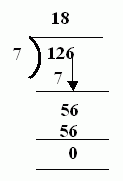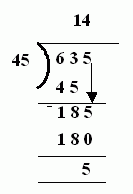Name: ___________________Date:___________________

 Email us to get an instant 20% discount on highly effective K-12 Math & English kwizNET Programs!

Grade 4 - Mathematics6.2 Division Problems

 Method: Read the questions carefully. Divide and write the quotient and remainder if any. Example: What is 126 divided by 7?Answer: 18R0 Example: What is 635 divided by 45?Answer: 14R5 Directions: Divide the following. Also write at least 10 examples of your own.
 Q 1: What is 1/3 of 3012201510 Q 2: What is 365 divided by 13?28R1280R127R52 Q 3: What is 603 divided by 17?36R1834R835R8305R8 Q 4: What is 22 divided into 479?21R2728R721021R17 Q 5: What is 436 divided by 18?24R424R724R4214 Q 6: What is 67 divided into 804?12R5221612 Q 7: What is 625 divided by 25?24R2513425025 Q 8: What is 15 divided into 615?434104140 Question 9: This question is available to subscribers only! Question 10: This question is available to subscribers only!